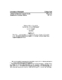## Backward Error Bounds for Approximate Krylov Subspaces##### Files
CS-TR-4247.ps(106.86 KB)
Let $A$ be a matrix of order $n$ and let $\clu\subset\comp^{n}$ be a subspace of dimension $k$. In this note we determine a matrix $E$ of minimal norm such that $\clu$ is a Krylov subspace of $A+E$. (Cross-referenced as UMIACS-TR-2001-32)